Measures of Skewness, Business Mathematics & Statistics

# Measures of Skewness, Business Mathematics & Statistics - Business Mathematics and Statistics - B Com

## Measure of Skewness

In statistics, we study about the management, observation and calculation generally over a large numerical data. In the statistical analysis of a survey or research, a researcher is required to know about the distribution, central tendency, dispersion etc.
It is also needed to know that what would be the variability and location of the given data set. This includes the measurement of skewness of the data, since all given data distributions are not symmetric.

Important

The skewness measures how asymmetric the distribution is. We can say that skewness is the measure of asymmetry of the data. It also determines if the data is skewed to the left or to the right.
The measure of skewness is being utilized in many areas. We know that a data which is normally distributed, is said to be symmetric about its mean. It has skewness equal to zero. But usually, the distributions are not symmetric.

Thus, the analysis of skewness becomes mandatory, as it defines the deviations from the mean position. An asymmetric or skewed data is not a perfect mirror image about the mean. By the measurement of skewness, one can determine how mean, median and mode are connected to one another. Let us go ahead in this page and learn about skewness, its calculation and applications in detail.

## Definition

Measure of skewness determines the extent of asymmetry or lack of symmetry. A distribution is said to be asymmetric if its graph does not appear similar to the right and to the left around the central position. In more statistical language, the skewness measures how much is the asymmetry of probability distribution of some given real-valued random variable about the mean. Skewness can be observed in the given data when number of observations are less.
For Example: When the numbers 9, 10, 11 are given, we may easily inspect that the values are equally distributed about the mean 10. But if we add a number 5, so as to get the data as 5, 9, 10, 11, then we can say that the distribution is not symmetric or it is skewed.
The skewness can be viewed by the having a look at the graph. The measure of skewness can be of two types : positive skew and negative skew.
Positive Skew: When the given distribution concentrates on the left side in the graph, it is known as the positive skew. In the following curve, we may easily observe that the right tail is bigger. This may be called as right-tailed or right-skewed distribution.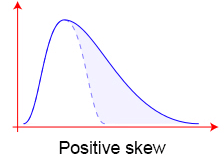Negative Skew: If in the graph, the concentration of the curve is higher on the right side or the left tail is bigger, then given distribution would known as left tailed or negatively skewed or left skewed. It is shown in the following diagram :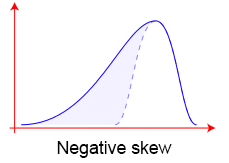## Formulas

There are various measures of skewness of the statistical data. For univariate data, the expression for measurement of skewness is given below: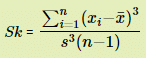Where, xi = given observations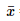= mean
s = standard deviation
n = number of observations.

For normal distribution, the measure of skewness is zero. When skewness is negative, it means that the data is left skewed. If it is positive, then the data is said to be right skewed.

Pearson’s Coefficient of Skewness

The method of finding skewness is also developed by Karl Pearson. This method is known as "Pearson’s Coefficient of Skewness". According to this method, there are two formulas for the measurement of skewness.
The formula is: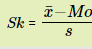Where, \$\bar{x} represents mean of the data, s is the standard deviation and Mo denotes the mode for the given sample.

This formula uses mode, thats why it is called "Pearson Mode Skewness".
There is another formula which utilizes median and eventually it is termed as "Pearson Median Skewness".
This formula is given below: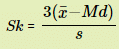Where, Md stands for median and all other symbols are as above.
These formulas are used according to the given values, i.e. when mode is known, then Pearson mode skewness is used and when median is given, Pearson median skewness is applied.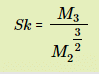Where,

M= Third moment of the given data and is calculated using the following formula: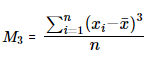and

M2= Second moment or variance of the data which is measured by the use of following formula :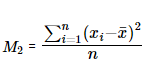## Applications

Measure of skewness is applied very commonly, since skewed data is seen quite often in different situations. In commerce, the skewness has to be measured very frequently when incomes are skewed to the right or to the left. On the other hand, the data which describes the lifetime of some commodity such as a tubelight, is right skewed. The smallest lifetime may be zero, whereas and the long lasting tubelights will provide the positive skewness to the distribution.

The document Measures of Skewness, Business Mathematics & Statistics | Business Mathematics and Statistics - B Com is a part of the B Com Course Business Mathematics and Statistics.
All you need of B Com at this link: B Com

115 videos|142 docs

## FAQs on Measures of Skewness, Business Mathematics & Statistics - Business Mathematics and Statistics - B Com

 1. What is skewness in business mathematics and statistics?Skewness is a measure of the asymmetry or lack of symmetry in a probability distribution. It quantifies how much a distribution deviates from being perfectly symmetric. In business mathematics and statistics, skewness helps to understand the shape and characteristics of data distribution, which can be useful in decision-making and analysis.
 2. How is skewness calculated?Skewness is typically calculated using the formula: Skewness = (3 * mean - mode) / standard deviation. This formula measures the difference between the mean and mode, scaled by the standard deviation. A positive skewness value indicates a right-skewed distribution, where the tail is longer on the right side. A negative skewness value indicates a left-skewed distribution, where the tail is longer on the left side.
 3. What does a positive skewness value indicate?A positive skewness value indicates a right-skewed distribution. This means that the majority of the data is concentrated towards the lower values, while the tail extends towards higher values. In business mathematics and statistics, a positive skewness may suggest that there are outliers or extreme values on the upper end of the distribution, impacting the overall shape and interpretation of the data.
 4. How does skewness affect data analysis in business?Skewness plays an important role in data analysis in business. It helps analysts understand the shape of the data distribution and identify any potential outliers or extreme values. Skewness can also impact statistical tests and models that assume a normal distribution. If the data is significantly skewed, it may be necessary to apply transformations or consider alternative statistical methods to ensure accurate analysis and decision-making.
 5. Can skewness be used to compare different datasets in business mathematics and statistics?Yes, skewness can be used to compare different datasets in business mathematics and statistics. By calculating the skewness for each dataset, analysts can determine which dataset has a more pronounced skewness and assess the differences in their distributions. This comparison can provide insights into the characteristics and patterns of the data, enabling better decision-making and understanding of business trends.

115 videos|142 docsExplore Courses for B Com examSignup to see your scores go up within 7 days! Learn & Practice with 1000+ FREE Notes, Videos & Tests.
10M+ students study on EduRev
Track your progress, build streaks, highlight & save important lessons and more!
Related Searches

,

,

,

,

,

,

,

,

,

,

,

,

,

,

,

,

,

,

,

,

,

,

,

,

;